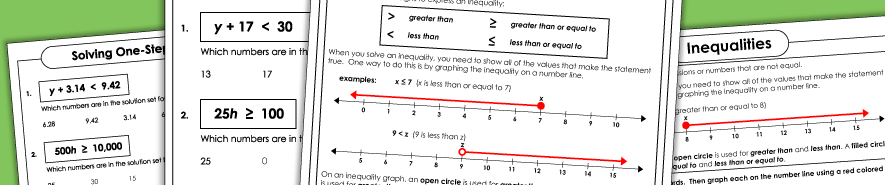# Inequalities WorksheetsSingle-Variable Inequalities (Basic)

Introduction to inequalities. Solve and graph the inequalities with only one variable. Students graph the inequality. They do not need to perform any steps to isolate the variable.
(example: a ≥ 7)

One-Step Inequalities

These inequality worksheets have one variable in each problem. Students need to isolate the variable using only one step.
(example: 12 - x ≤ 8)

More Algebra Worksheets

This page has very basic worksheets to help students identify dependent and independent variables, evaluate algebraic expressions and solve for variables in equations.